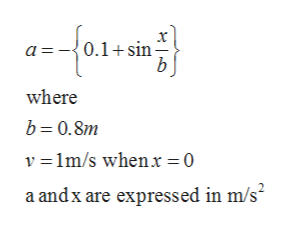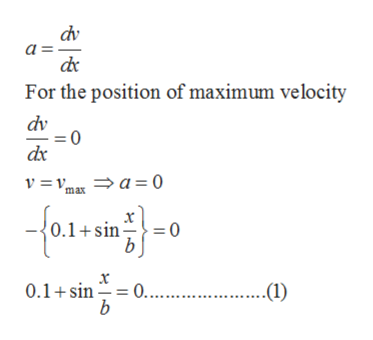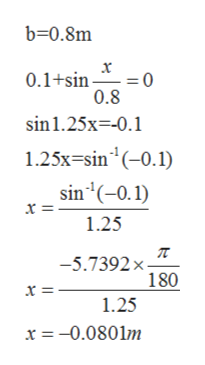Based on experimental observations, the acceleration of a particle is defined by the relation a = –(0.1 + sin x/b), where a and x are expressed in m/s2 and meters, respectively. Know that b = 0.80 m and that v = 1 m/s when x = 0.Determine the position where the velocity is maximum.

Question

Based on experimental observations, the acceleration of a particle is defined by the relation a = –(0.1 + sin x/b), where a and x are expressed in m/s2 and meters, respectively. Know that b = 0.80 m and that v = 1 m/s when = 0.

Determine the position where the velocity is maximum.

Step 1

The acceleration of the particle is given as the:help_outlineImage Transcriptionclose0.1+sin where b 0.8m v 1m/s whenx 0 a andx are expressed in m/s2 fullscreen
Step 2

The maximum velocity of the particle will be when acceleration of the particle is zero

As we know:help_outlineImage Transcriptionclosedv a For the position of maximum velocity dv dx v = v, a 0 ma 0.1+sin 0 = x 0.... b 0.1 sin ..(1) fullscreen
Step 3

Put the value of b in the equat...help_outlineImage Transcriptioncloseb-0.8m x 0.1+sin 0 0.8 sin1.25x-0.1 1.25x-sin(-0.1) sin'(-0.1) x = 1.25 -5.7392 x x = 180 1.25 x = -0.0801m fullscreen

Want to see the full answer?

See Solution

Want to see this answer and more?

Our solutions are written by experts, many with advanced degrees, and available 24/7

See Solution
Tagged in

Mechanical Engineering